Education ∪ Math ∪ Technology

# Month: April 2013(page 1 of 2)

At our school we currently have open-access to the printer by our students. Unfortunately our students waste a lot of paper, usually reprinting something 10 times without checking to see if the printer is actually working and/or has enough paper. We work with students to try and improve their use of the printer, but in the mean-time, we have a lot of paper which has already been printed on one side, and which would otherwise go to waste.

Here are some ideas on what schools could potentially do with all of that paper.

1. Use it for paper folding.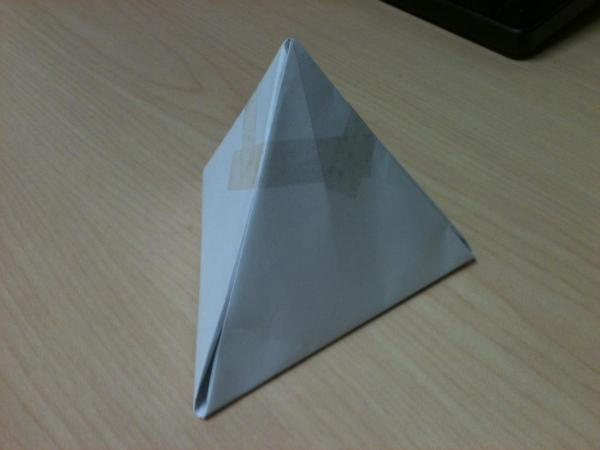Paper folding has strong links to mathematics and art. It could provide a context for a mathematical activity so that students see the mathematics they are learning applies to parts of the world, rather than being just symbols on paper. Students can also use paper folding as a way of expressing themselves through art.

2. Students and teachers can obviously use the extra paper as rough paper for writing, drawing math diagrams, or a whole bunch of other things.

3. Send the paper to a school in need. Our partner school in Kipevu, Mombassa, Kenya typically runs out of paper half-way through a semester if they are lucky.

4. Some of the paper may have information on it which is sensitive in nature. You can first send the paper through a paper-shredder, and then use it for weaving, which can be handy when you need baskets around the school. What school wouldn’t benefit from having more storage containers available?(Image credit: josey4268)

5. Flip it over and print on it again. The only problem here is that printers are typically more likely to jam with paper that has already been used, so this may be a problem, depending on your model of printer.

6. Use it to line the inside of animal cages in your science department.

7. Cut it into smaller rectangles and use them for exit slips.

8. Turn the paper into envelopes and have students send "Get well soon" and "I miss you" messages to their classmates that are sick, or to their penpals in another school.

9. Turn the paper into paper airplanes and have an iterative design contest. The objective of this type of contest is to collectively build the best paper airplane you can by cycling between creating paper airplanes, observing how well they fly, and then redesigning the airplanes. From this activity students will learn that design is messy, collaborative, and fun.

Any other suggestions?

I’ve started a presentation on grading, which anyone with the link can edit and add their perspective. The presentation is split into four areas:

• What are the goals of grades?

• What evidence supports the use of grades in schools?

• What evidence does not support the use of grades in schools?

Please feel free to add your perspective to this summary of the debate on grading, ideally citing evidence for any strong claims that you make if possible.

Remember that there are a lot of emotions behind the different perspectives on this issue, so to refrain from personal attacks, etc… My objective is to gather evidence and perspectives, rather than start any flame wars.

My mother has offered to give us a small amount of money, which we can either use to pay down most of our debt, or we can put into a savings account, and then pay down the debt over time.

My wife and I have been debating which is the better course of action. After playing around in Excel, I decided to write a script to help us resolve this issue. See https://davidwees.com/javascript/debtsavings/

I also gave this problem (with the actual numbers my wife and I are looking at) to my 12th grade students as part of our review for their IB exams in May. One student’s reaction, "This is a real problem? Really? Wow."

*Disclaimer: The script in question is a work in progress, it may contain bugs, and should not be relied upon to do any actual financial planning.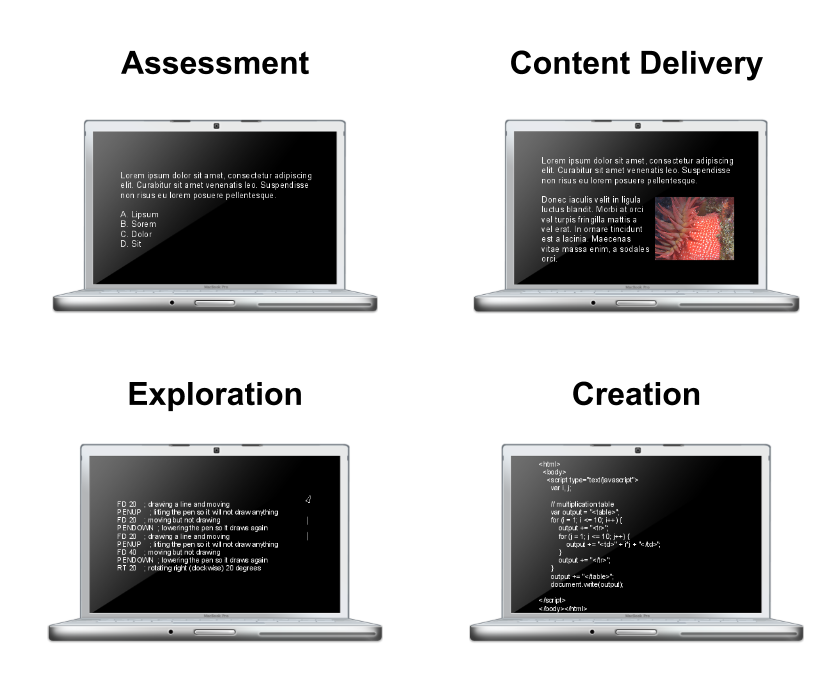Which of these ways dominates? Why?

* Note: There are some tasks which overlap. Programming a computer (under the creation category) to achieve a specific task could be a form of assessment, for example. Also, one could probably argue that "exploration" and "programming" aren’t really distinct categories.

"Just as we know students don’t learn simply because we tell them something, teachers don’t learn simply because we hand them a journal article." ~ Jo Boaler

This is part of my daily challenge as a learning specialist for mathematics and information technology. I can share research, suggestions, and resources, but how much of what I share actually changes practice?

Here is what I have found that does make a difference in their learning for my colleagues:

• I develop strong positive relationships with my colleagues,
• I share timely resources with my colleagues,
• I help them plan units and lessons,
• I work with my colleagues in one on one sessions and offer them support that meets their current needs,
• I share the big picture and my vision with my colleagues (and listen to their vision to make it our vision),
• I visit their classes and give them support (as opposed to enforcing a specific direction) in the context of their classroom practices.

Here’s what I do not do:

• I do not make my colleagues feel stupid, even when I am showing them how to do something for the 5th time,
• I try my best not to preach,
• I don’t keep what I do to fix their technology problems a secret,
• I try not to overwhelm my colleagues with too many changes at once,
• I do not ignore their requests for help.

Grant Wiggins shared an article on his blog called "The Nature of Proof." The article describes a course in geometry given in the 1930s that was not only extremely influential for those who took the course, many of them described it as the most important course they ever took in their entire lives. Here’s a quote from the teacher of that course.

"While teachers of mathematics say they want the young people in our secondary schools to understand the nature of proof, that should not be and probably is not their total concern. What these teachers really want is not only that these young people should understand the nature of proof but that their way of life should show that they understand it. Of what value is it for a pupil to understand thoroughly what a proof means if it does not clarify his thinking and make him more "critical of new ideas presented"? [emphasis mine] The real value of this sort of training to any pupils id determined by it effect on his behavior and for purposes of this study we shall assume that if he clearly understands these aspects of the nature of proof his behavior will be marked by the following characteristics:

1. He will select the significant words and phrases in any statement that is important to him and ask that they be carefully defined.
2. He will require evidence in support of any conclusion he is pressed to accept.
3. He will analyze the evidence and distinguish fact from assumption.
4. He will recognize stated and unstated assumptions essential to the conclusion.
5. He will evaluate these assumptions, accepting some and rejecting others.
6. He will evaluate the argument, accepting or rejecting the conclusion.
7. He will constantly re-examine the assumptions which are behind his beliefs and which guide his actions."

Harold Fawcett, The Nature of Proof, Page 11, 1938

The use of "He" in this quote should be understood in the context of when this quote was written, and could easily be substituted for "The student" or something similar.

My previous article focused on using programming as an example of applying math one already knows to a different context. The purpose of this article is to describe how one could, through programming, learn a new mathematical concept.

What I will do is use some examples to show one could start with an exploration using Turtle Art (which is a programming environment designed for kids) and use this exploration to end up developing models of how a few areas of mathematics work. You can download the examples here and open them up in Turtle Art to explore them yourself.

In Turtle Art, programming is done through the use of snappable blocks, each of which represents a different programming structure. It is very similar to the Scratch programming environment, both of which are based off of the Logo programming language. Any decent exploration of Turtle Art should start with attempting to snap different blocks together and see what they do.

You could show students how to use one of the simplest blocks, the forward block. The output of this block is shown below.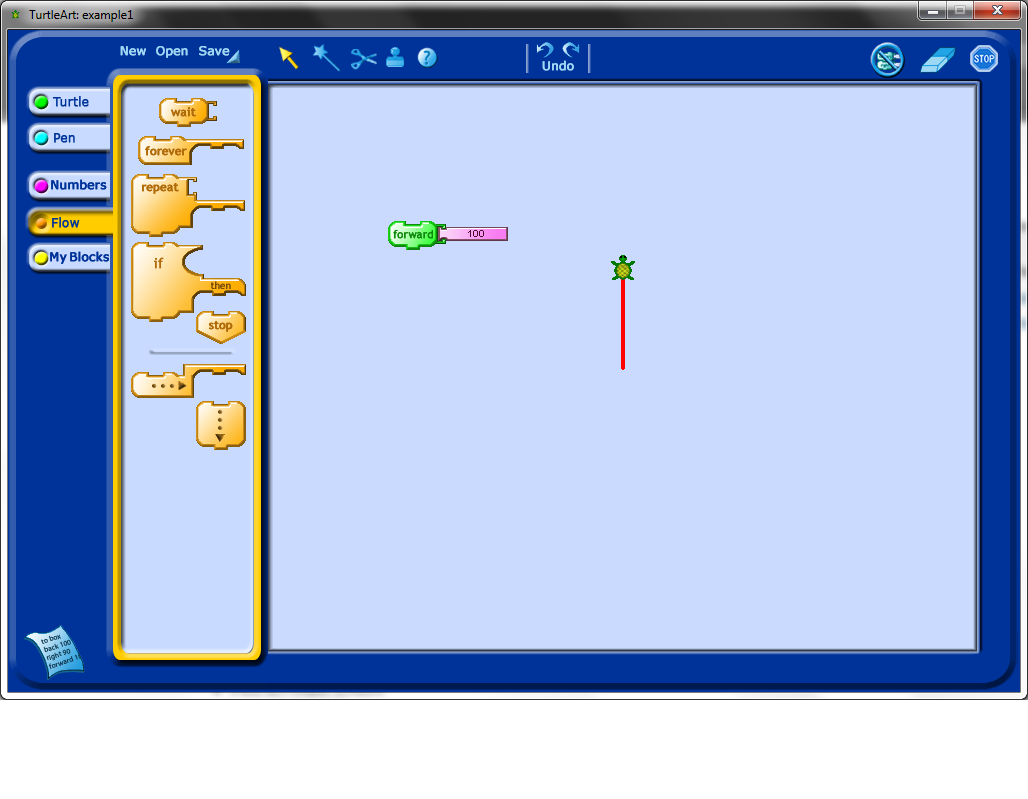Line segments are most interesting when attached to rotations, so the next block worth showing students is the rotation block. Note that as in the picture below, creating a program with these blocks means attaching them together and double-clicking on the paired blocks to see the output of your program.

Notice that, in the example below, the output is very similar as the first example, except that the turtle icon is rotated now 90 degrees relative to where it used to be.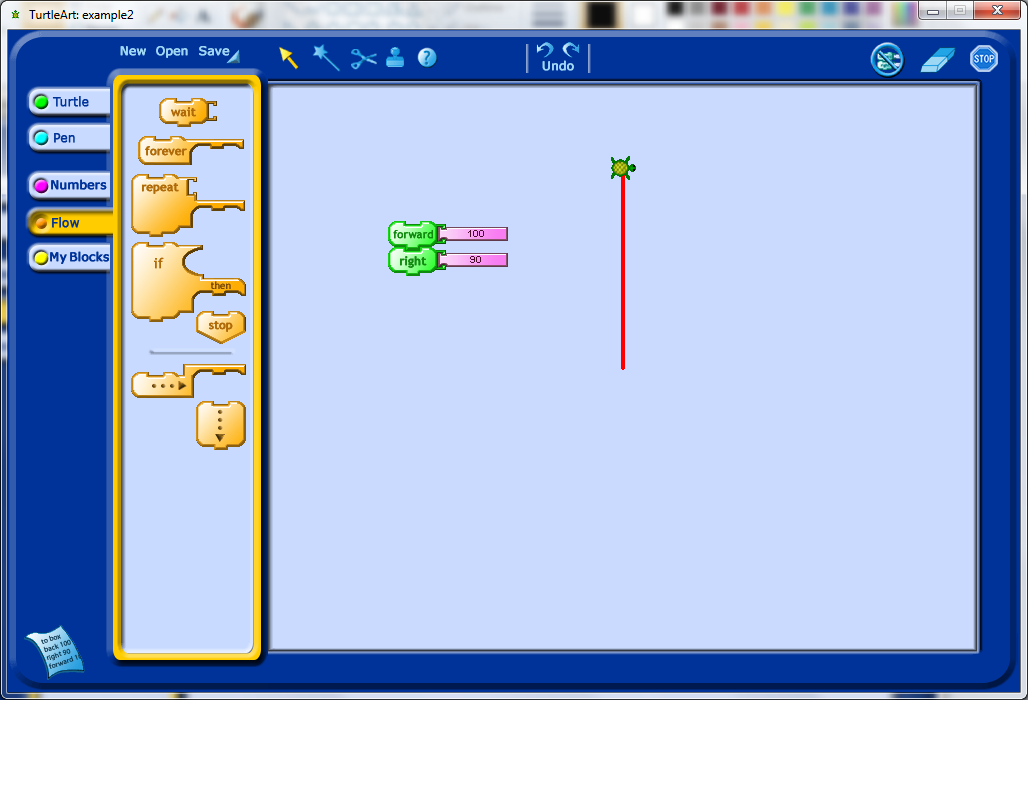Once students have played with rotations and moving forward, it is probably time to show them how to use the repeat function. The repeat function is one of the more powerful commands in Turtle Art as it allows any subcommands placed inside it to be replicated exactly any number of times.

Notice how you could add a square to your picture at this stage by using the same commands as before, but repeated four times. This suggests that a property of squares is that they can be generated from repeating the steps "forward n, rotate 90 degrees" four times.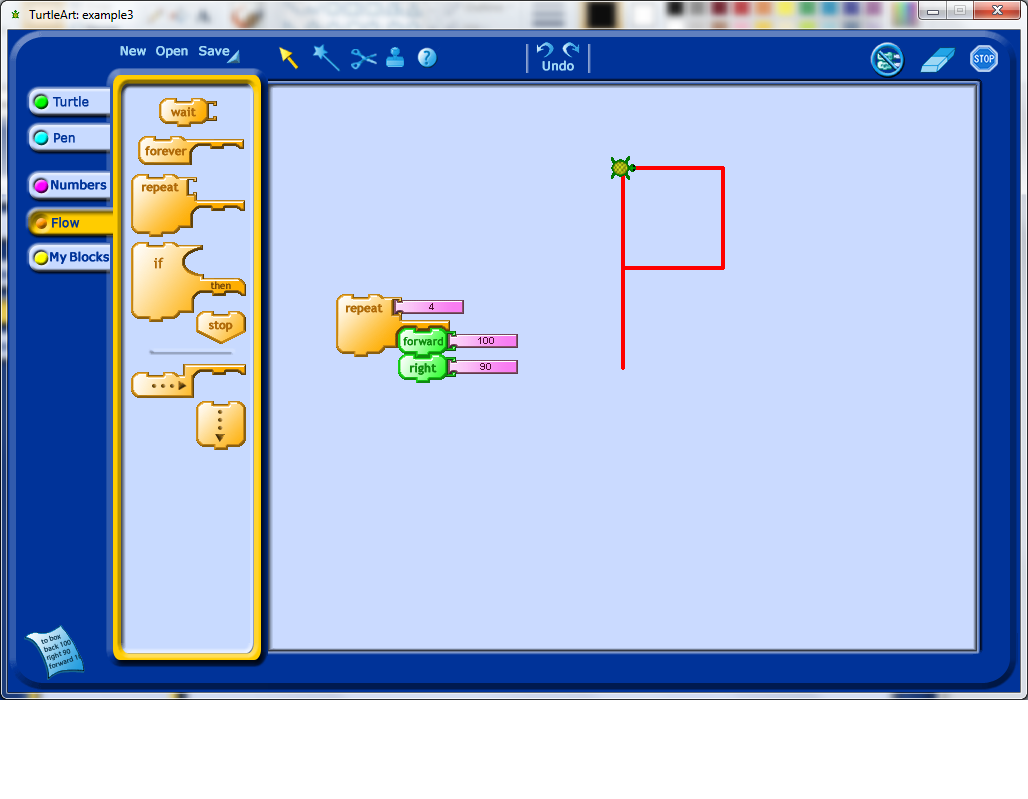At this point, students may realize that it would be convenient to start fresh each time, and so you can point out the clear block. Note that this block also resets the default starting position of your turtle.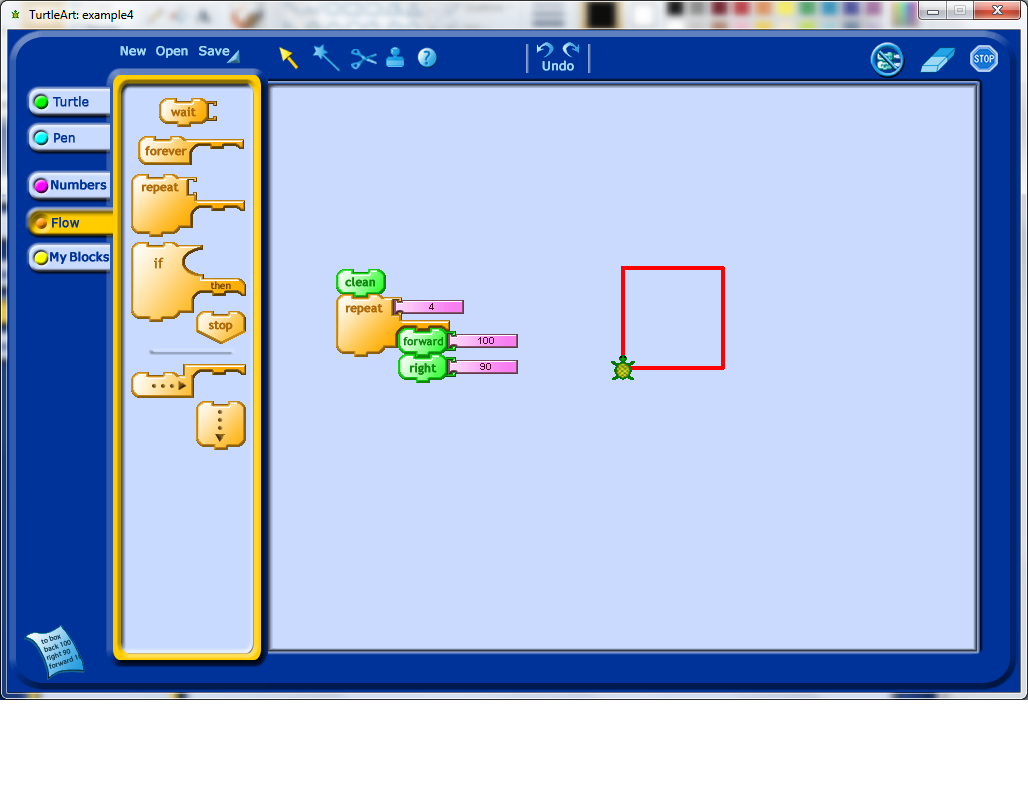One thing I’ve noticed students do very early on, when they explore Turtle Art programming, is change the angle of rotation. I’ve changed the angle from 90 degrees to 30 degrees in my example, and now all of the shape isn’t a square anymore.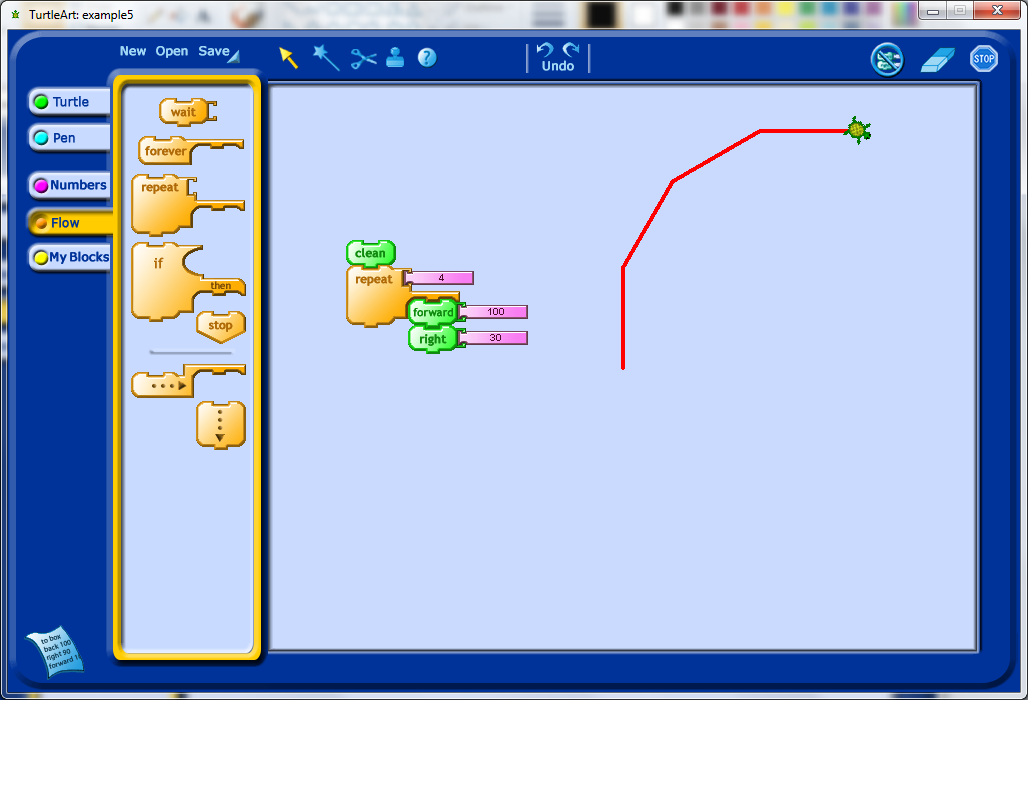After this, it would be natural to try and figure out how to close the shape one has drawn. Students might realize right away how many rotations they need, and they may need to experiment a bit. This experimentation, along with other related experiments that they will do, may lead them to develop a model for the number of degrees of rotation and how many repetitions of their initial forward plus rotation block they need. Below, pretending that I was a student, I only repeated the commands 8 times.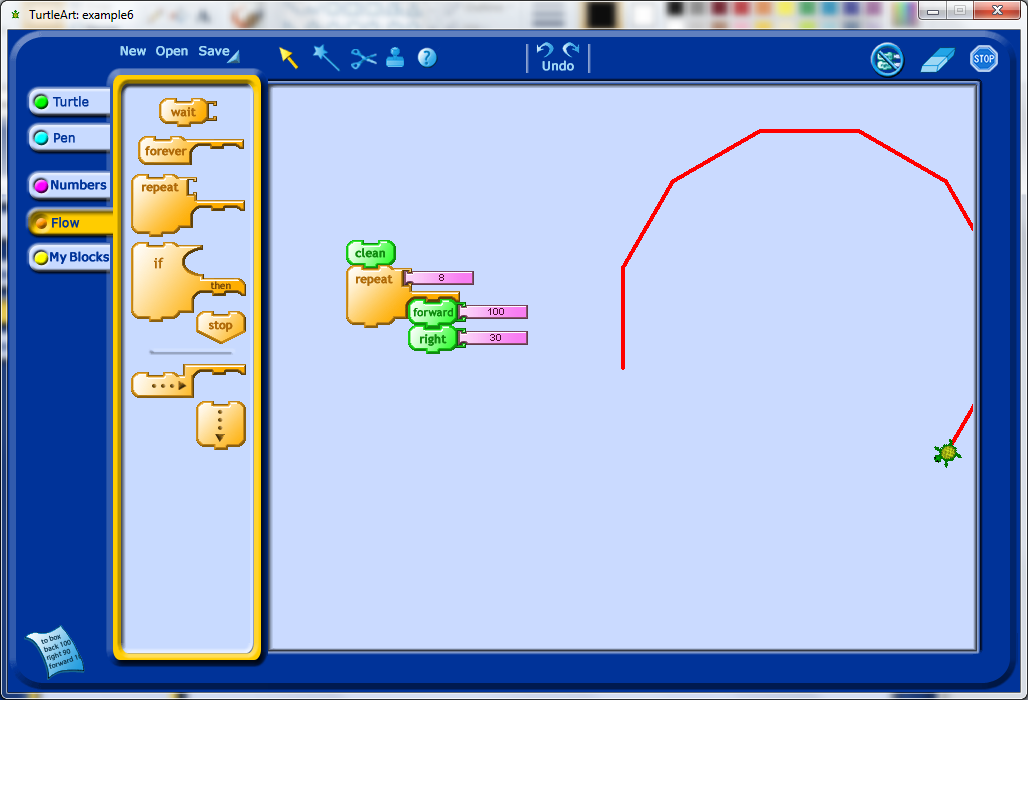When you see your students have made this kind of discovery, it may be worth checking in with them to see if they have a model in mind for why 12 repetitions worked, and why 11 or 13 would not. In particularly, it would be interesting for them to explore, for example, what happens if they change the angle of rotation? What happens if they change how far they go forward each time?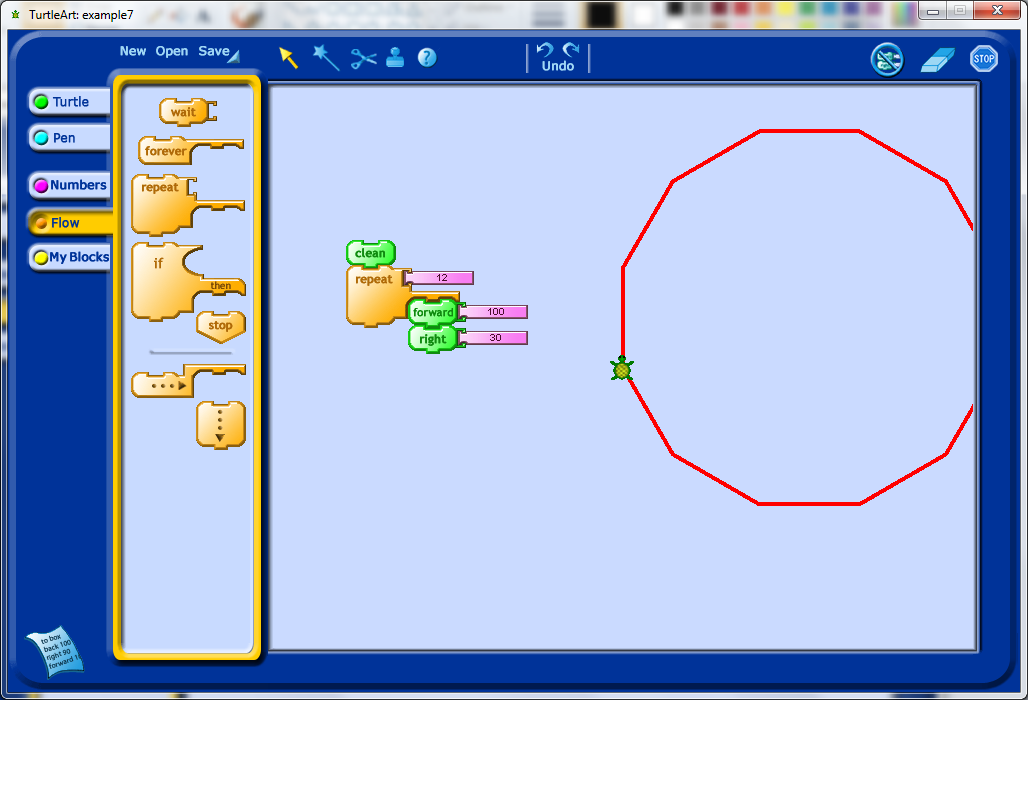Another thing that I have noticed students often do, once they learn about the repeat block, is stack repeats to see what happens. In this case, the extra repeats do not appear to have changed the output at all, which should hopefully lead to some questions by the student. I would recommend having the students step through the program one command at a time and see if they can understand the reason why the output is what it is.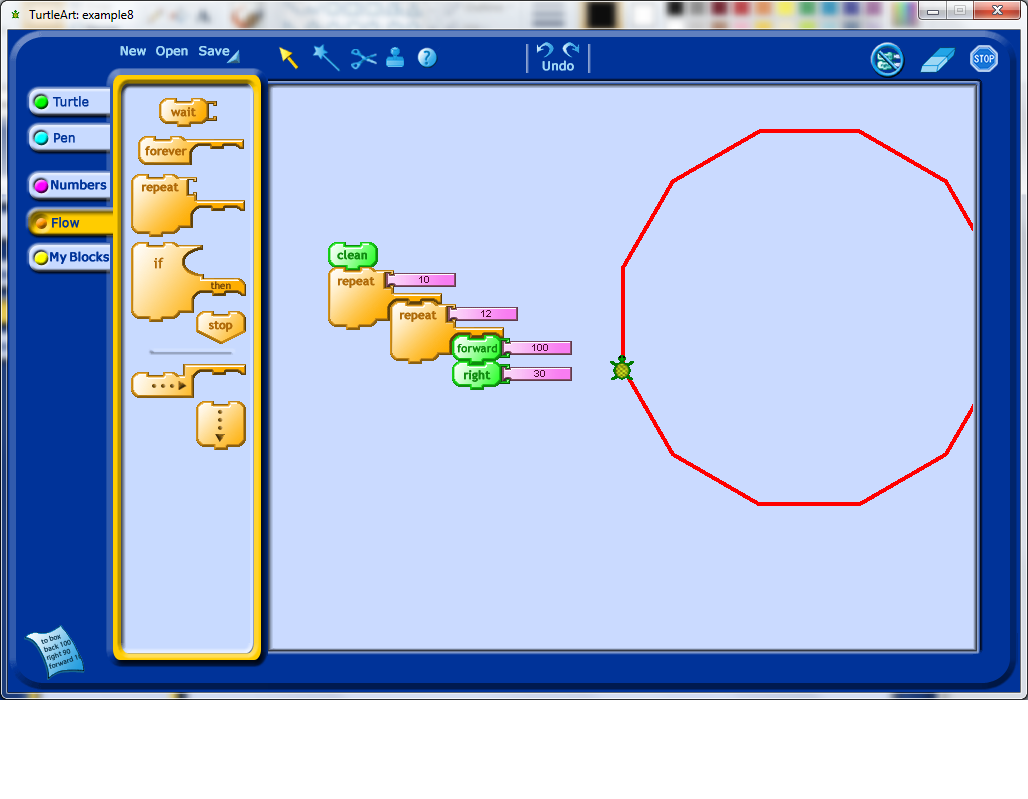Having understood the issue in the previous step, I decided to add a forward and a rotation into my double repetition, which leads to a fairly interesting shape which unfortunately extends beyond the edges of the view window set by the program.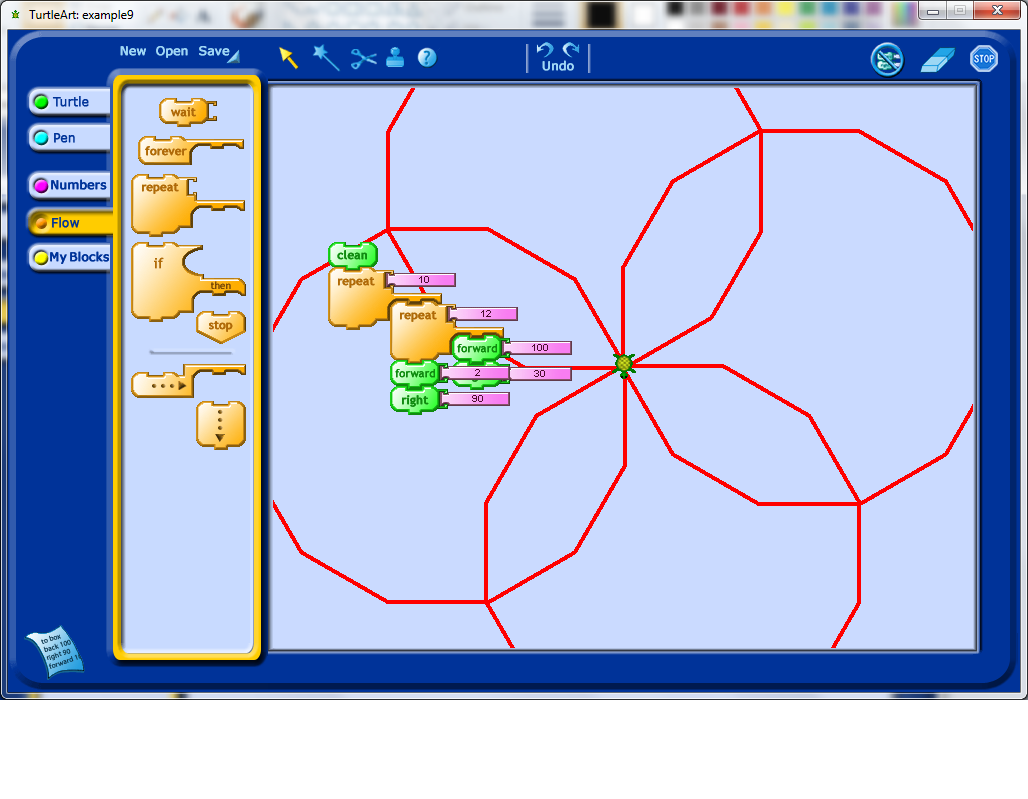At this stage, I decided to figure out how to shrink my shape. This, with some prompting, could lead to a discussion about variables. With some experimentation, students may learn that variables are an input into the program they have created which may affect the output of the program.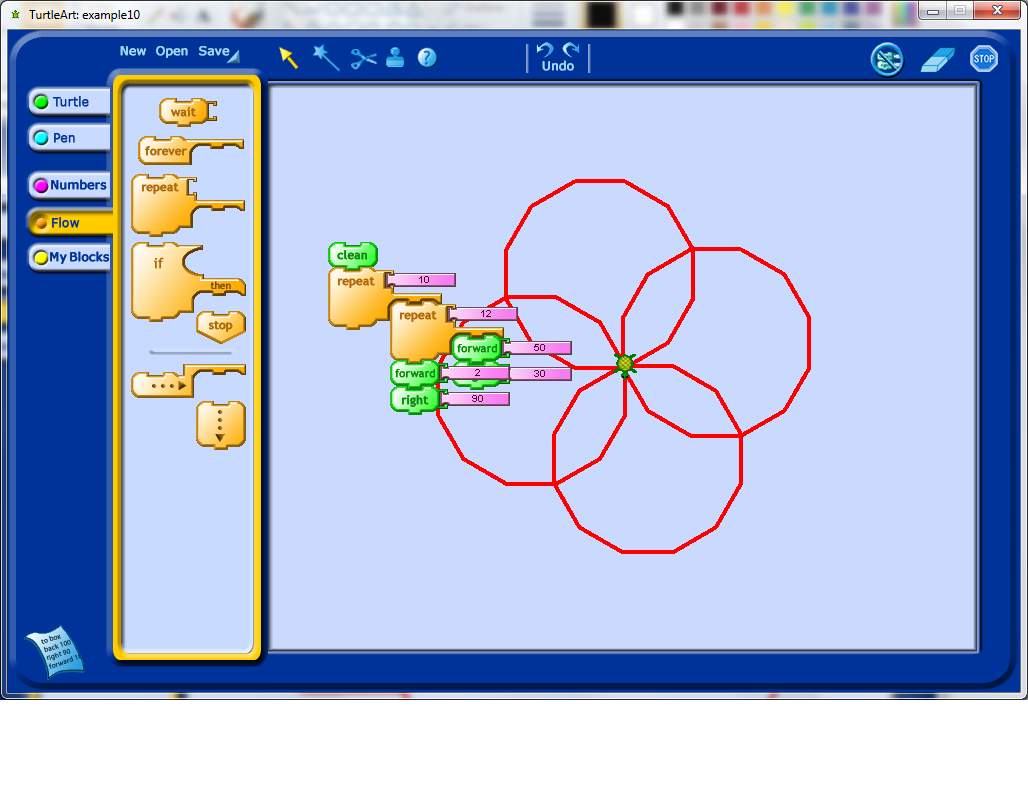My first instinct after seeing the rotation above was to change the second angle of rotation to only 10 degrees to see how this influenced the output. Right away I decided that I would need to change the number of repetitions for the outside repeat loop. I would encourage any students working on an activity like this to play around with changing the number of repetitions to see if they can figure out how many repetitions will make their pattern repeat all the way around a complete revolution.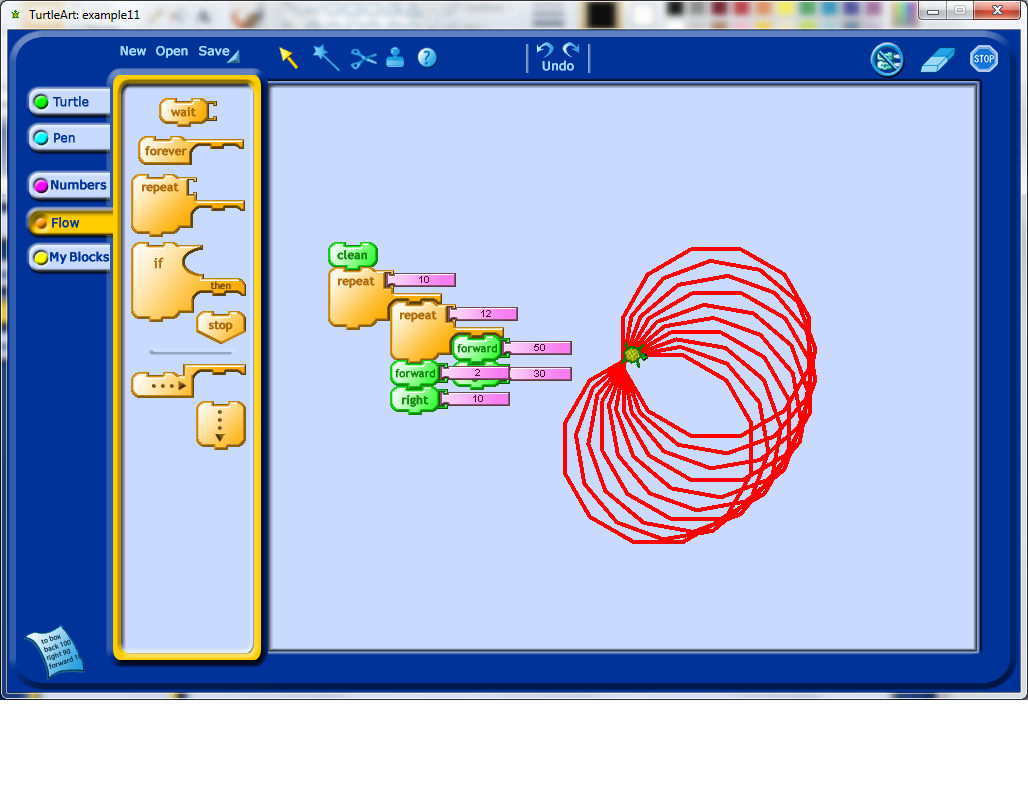Now we really have an interesting looking shape. Notice that to get this shape, we repeated our outside loop exactly 36 times. Why 36 times, why not another number? What would happen if used a larger number?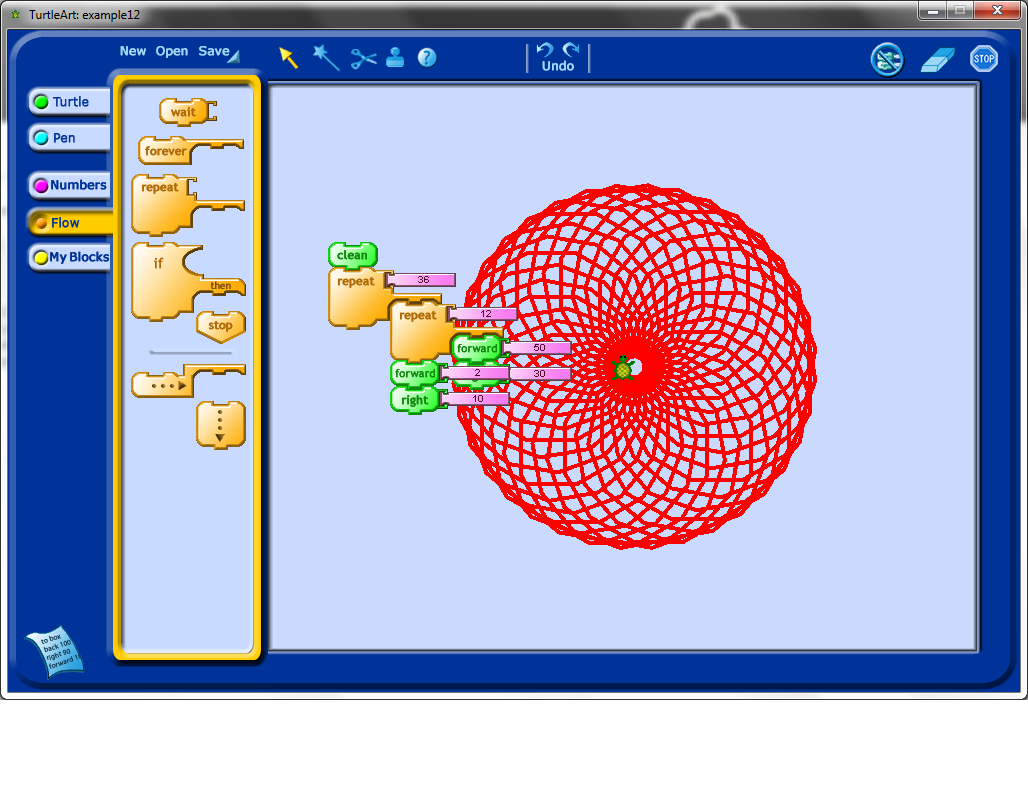I found the Turtle icon sitting in the middle of the other picture to be a bit distracting, so I tried to use setxy (which, by the way, students absolutely find hysterical if you mispronounce it) to move it. Notice that this particular code block would lead to a discussion about Cartesian coordinates, and that through experimentation, especially if students have an understanding of negative numbers already, lead to understanding the purpose of a coordinate system.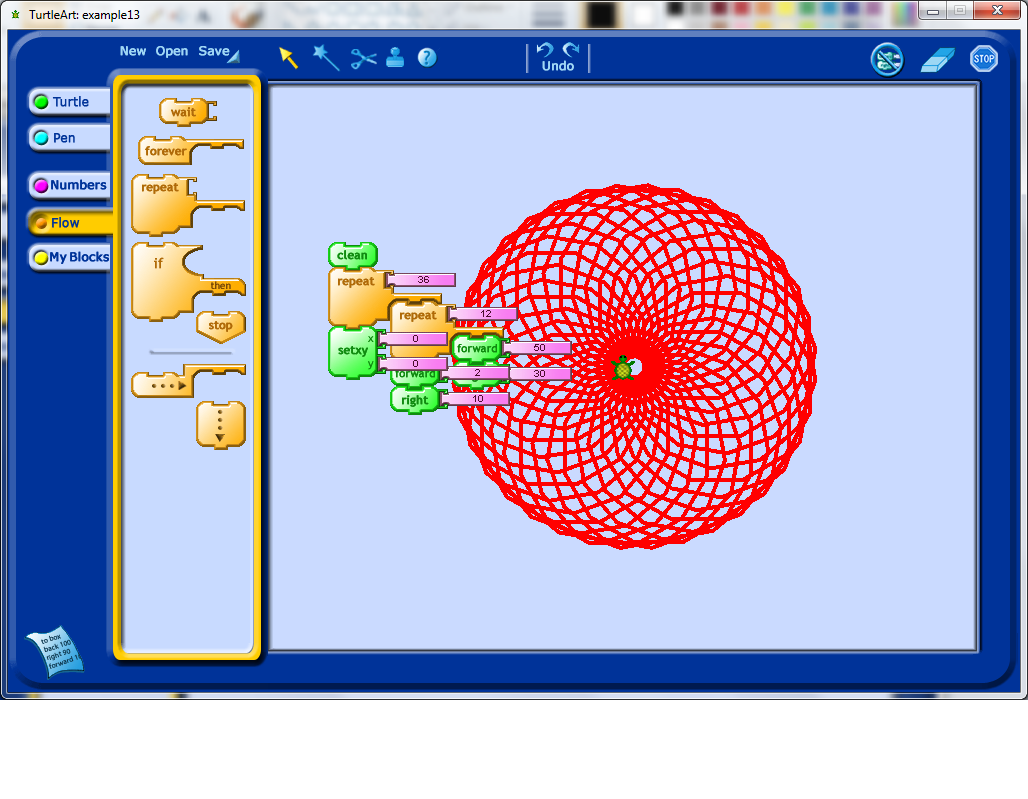I did some further exploration with the setxy tool, trying to get the Turtle icon off of my shape. Note that actually determining the actual size (in pixels) of this shape involves using trigonometry, so one could turn any exploration of shape into a mathematics problem session as well, simply by asking students to try and work out the sizes of the objects they create.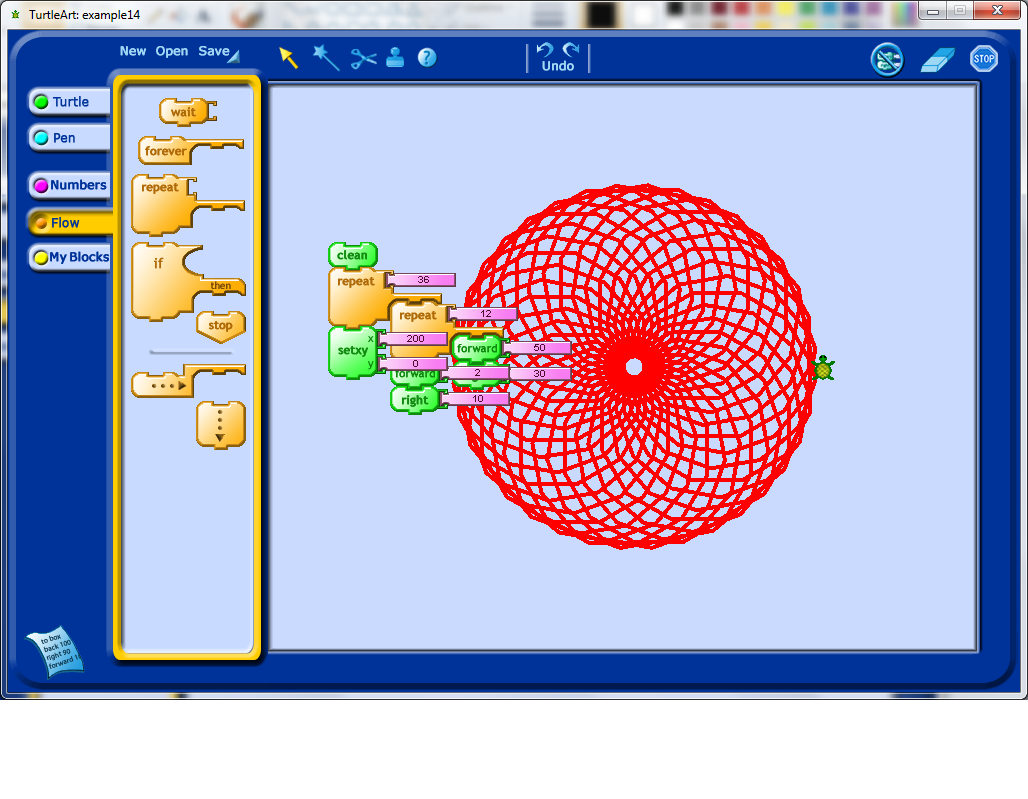I finally got the Turtle off of the shape.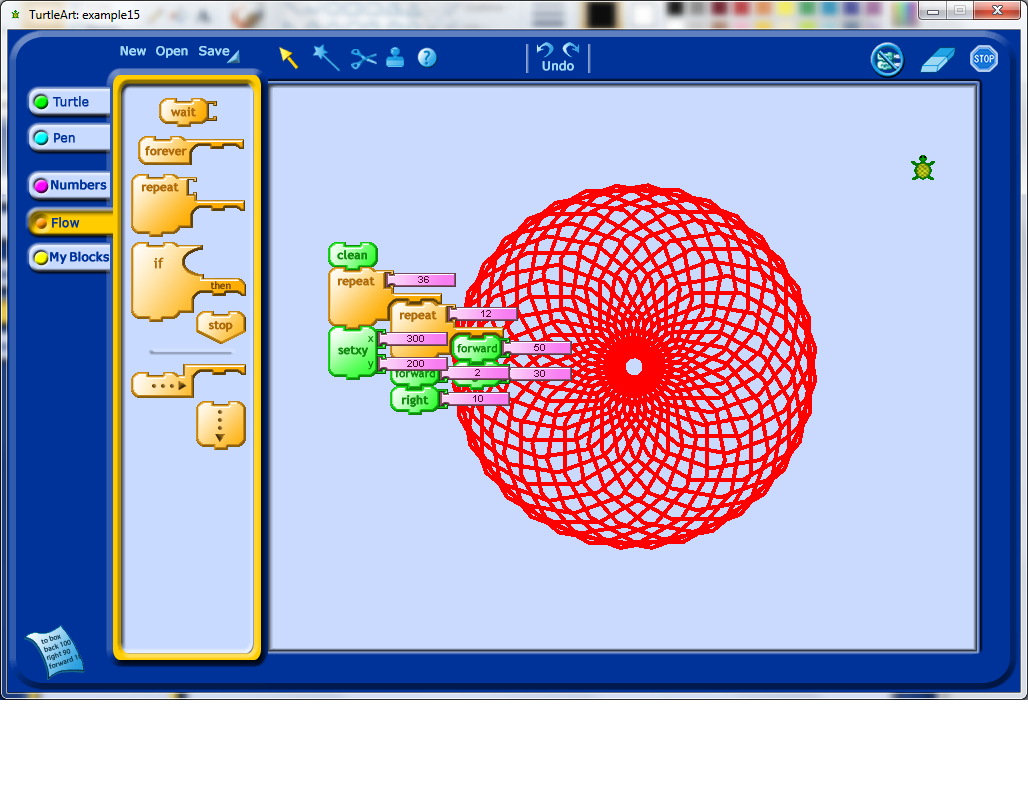I decided that it would be nice to draw another one of these shapes in the new Turtle location, which meant I had to start changing my code to more easily allow for repetition of the code structures. Turtle Art allows for the person using it to define their own blocks. In this case, I decided to create a procedure called "Shape" which would be used to draw just the original 12 sides shape. I then called this procedure from the main block of code (see below).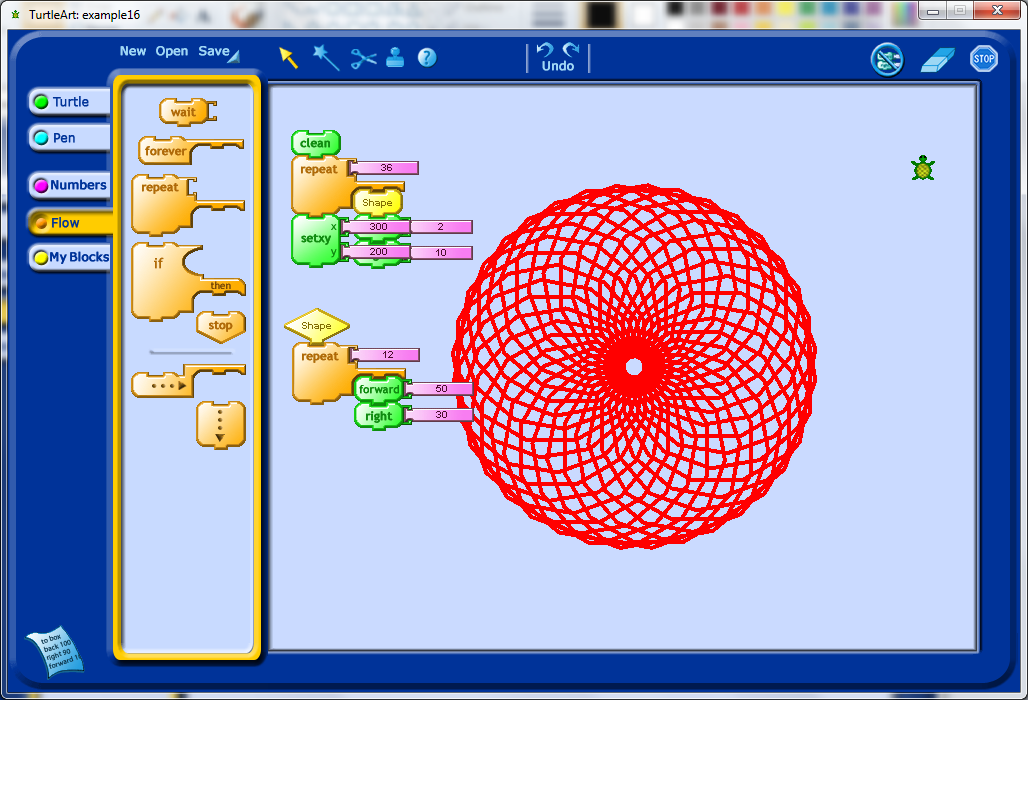I then decided to make the put the rest of the code into it’s own block as well, which I called Flower. If you look below, you’ll see that I first clean the screen, move myself into the middle of the screen, draw the flower, move to the edge of the screen, and redraw the flower shape. It occurred to me that it would be nice to change the size of the flower if necessary. Doing this would require introducing a variable.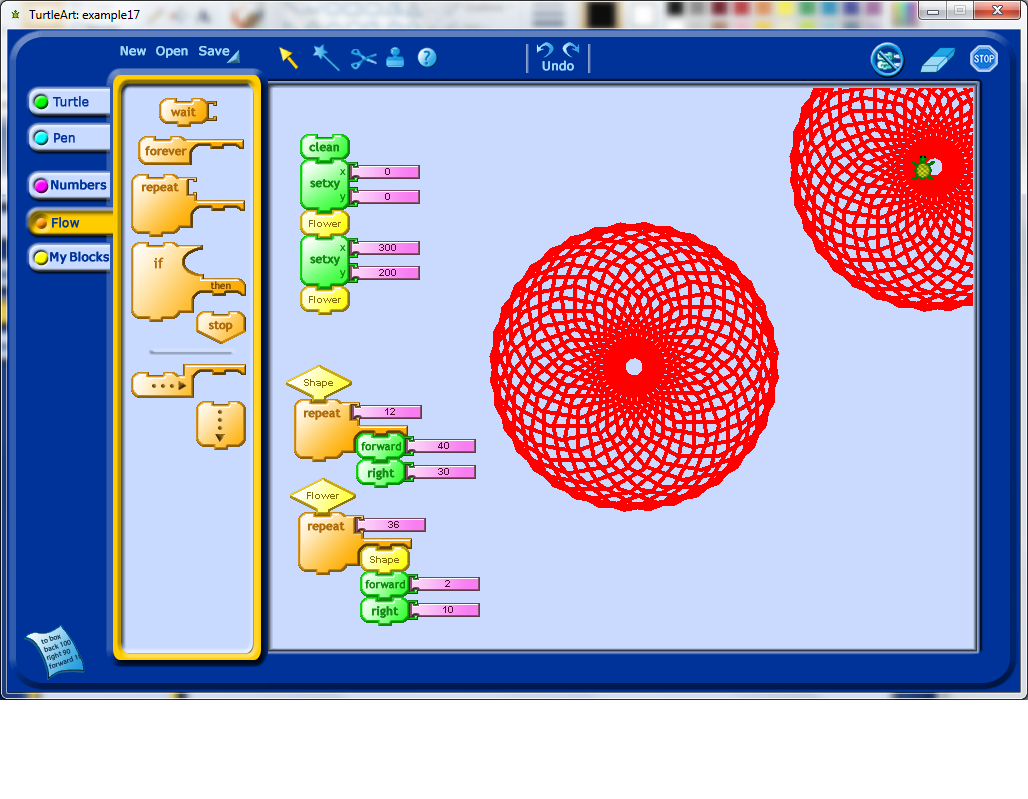I created a variable, which got called box1 (I wonder if I can rename this somehow). I set the value of box1, and then call the Flower procedure, and inside the Shape procedure, the value of box1 is used to determine the amount of forward movement done by the Turtle icon while it draws the Flower shape. To draw a flower of a different size, I changed the value of box1 again, and then called the Flower procedure again.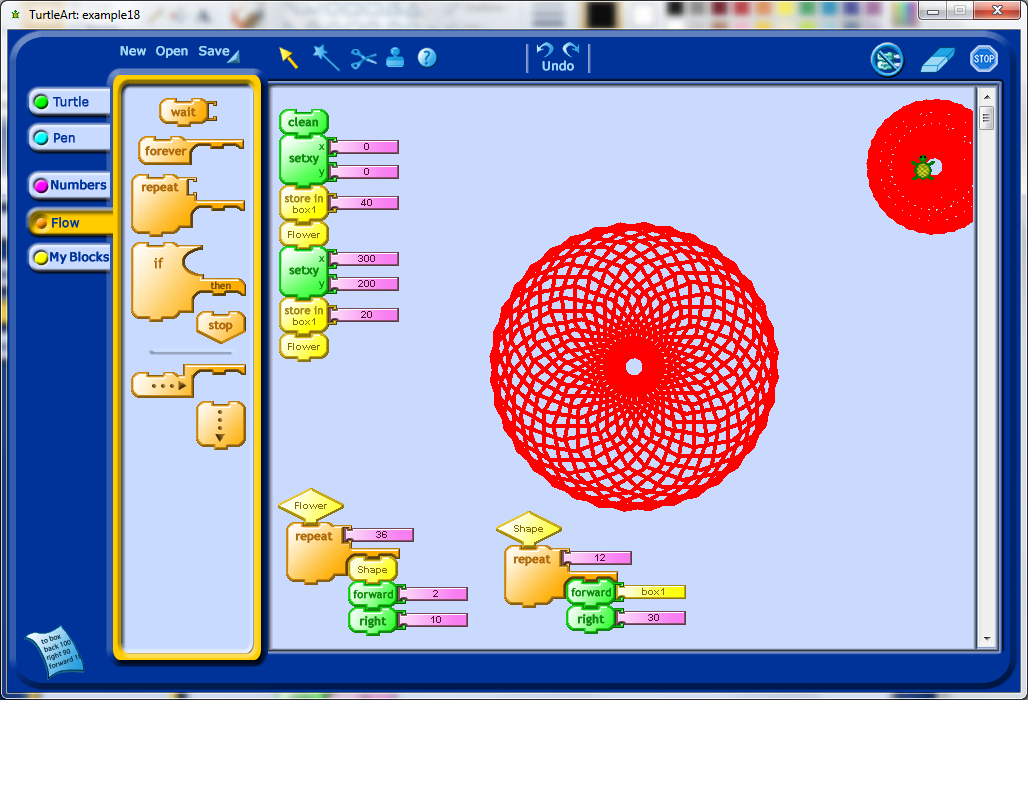You should see that even in a relatively simple example, there are a lot of embedded mathematical concepts. Through trial and error, careful observation, and a teacher’s mentorship, students should be able to develop some models of how these mathematical ideas work, within the Turtle Art environment. Besides learning mathematics, students will also have to develop other habits, such as patience, and the willingness to trouble-shoot and debug their programs.

The purpose of this post is to show an example of using math in the context of programming. I’ve written the post as I created the project, to try and outline my thinking during this process as much as I can. This is an example of me applying math that I know to a different context, creating a spiral of numbers. In a future post I plan on exploring what learning new mathematics through programming could look like.

Note: This post will look much better in the online version if you are reading it in an RSS reader or your email.

First, I start with a pretty basic HTML file with some an embedded JavaScript library called jQuery, and a blank Canvas HTML element.

[html5]

Prime Spiral

[/html5]

// init()
$(document).ready(function () { }); // debugging function function debug(message) {$(‘#debug’).append(message + ‘
‘);
}

So far all I have done is create two template files (which I always create when I am starting a new project written in JavaScript), and I have added the minimum skeleton to both of these files to get my project started.

// settings
var settings = {};
settings.numberOfNumbers = 200;

At the beginning of the JavaScript file, I add a settings variable. This will allow me later to tweak my project settings in one central location, rather than having to find where I have an embedded setting and modify it.

Next, I decided to resize the canvas so that regardless of what browser will view the canvas, it will be visible in their browser window.

// resize the canvas to the minimum of height and width for the screen
function resizeCanvas() {

// get the new width of the canvas, which should be the minimum of the height or width of the page
settings.dimension = $(window).height() >$(window).width() ? $(window).width() :$(window).height();

// now shrink it slightly
settings.dimension = settings.dimension * 0.90;

// now resize the canvas elements
$(‘#theContainer’).css({‘height’: settings.dimension, ‘width’: settings.dimension});$(‘#theCanvas’).css({‘height’: settings.dimension, ‘width’: settings.dimension});

}

There are two pieces of mathematics here. One is a comparison between the height of the browser window and it’s width, and the other is shrinking of the region to 90% of the original dimensions. I could have used the built in minimum value function for the first part, but for some reason, I chose not to.

The next step is to actually write the numbers in a spiral. To do this, I decided to work in polar coordinates, because spirals are convenient to draw in that coordinate system, but unfortunately browsers operate in Cartesian coordinates. Each point in the browser window can be labelled with an x, y position, as measured from the bottom-left corner of the canvas. My objective is to start numbering the spiral from the center of the canvas, so I will have to translate each point, after having converted from polar coordinates to rectangular coordinates.

Here is the function for the conversion:

// convert from polar coordinates to offset cartesian coordinates

// A point at (radius, theta) can also be thought of as being
// at the end of the hypotenuse of a right-angled triangle,
// hence we can use trigonometry to find the Cartesian coordinates.
// Note: theta will need to be in radians to be useful here, since
// the Math.cos() and Math.sin() functions assume the angle is in radians.

// now shift the point into the center of the canvas
x = x + settings.dimensions/2;
y = y + settings.dimensions/2;

// return both points as an array
return new Array(x, y);
}

Now comes a moment of truth. I have not yet been able to test these most recent functions I have created, so they may have mistakes in them. I need to actually attempt to use these functions to find out if they return the expected values. My next step is to therefore try and produce a function which will create the spiral of numbers, using the functions I have so far as tools. First, I want to cycle through all of the numbers, which looks like this:

// draw the spiral of numbers
function drawNumbers() {

// cycle through each number from 1 to the maximum number of numbers given
for (var index = 1; index <= settings.numberOfNumbers; index++) { } }

This is essentially a sequence of numbers where I start with a value of 1, and I increase them (using index++), until I reach the maximum value as set globally in the script.

With each of these numbers, I am now going to want to calculate what it’s position would be, remembering that I’m going to work in polar coordinates. I want the radius of the position of the numbers to slowly increase in a linear way, from an initial value of zero, to a maximum value of just less than half of the maximum width of the canvas. Therefore when the number is 1, this should map to a radius of zero, and my maximum number should map to my maximum radius. Here’s my initial guess as to what this conversion should be:

radius = (index – 1) / settings.numberOfNumbers * (settings.dimension / 2) * 0.90;

For the angle, I just want it to increase at a constant rate for each number, remembering that I will be working in radians. An increase in angle of $\frac{1}{32}$ of a full rotation of a circle seems like a reasonable initial guess.

theta = (index – 1) * (1 / 32) * Math.PI;

Next, I used the function I created earlier to convert into Cartesian coordinates (with the shift), and finally I created a function which should actually display the numbers on the canvas. Here is what the full spiral function looks like at this stage:

// draw the spiral of numbers
function drawNumbers() {

// define the variables

// cycle through each number from 1 to the maximum number of numbers given
for (var index = 1; index <= settings.numberOfNumbers; index++) { // set the radius so that it increases as the index increases, but is initially zero // note that we also want to scale the radius down slightly so that our spiral // is slightly smaller than the canvas radius = (index - 1) / settings.numberOfNumbers * (settings.dimension / 2) * 0.90; // our angle should increase at a constant rate, starting at 0 theta = (index - 1) * (1 / 32) * Math.PI; // now convert these into Cartesian coordinates coordinates = convertFromPolarToCartesian(radius, theta); // and then actually draw this number drawNumber(index, coordinates.x, coordinates.y); } }

Here’s the output of this function.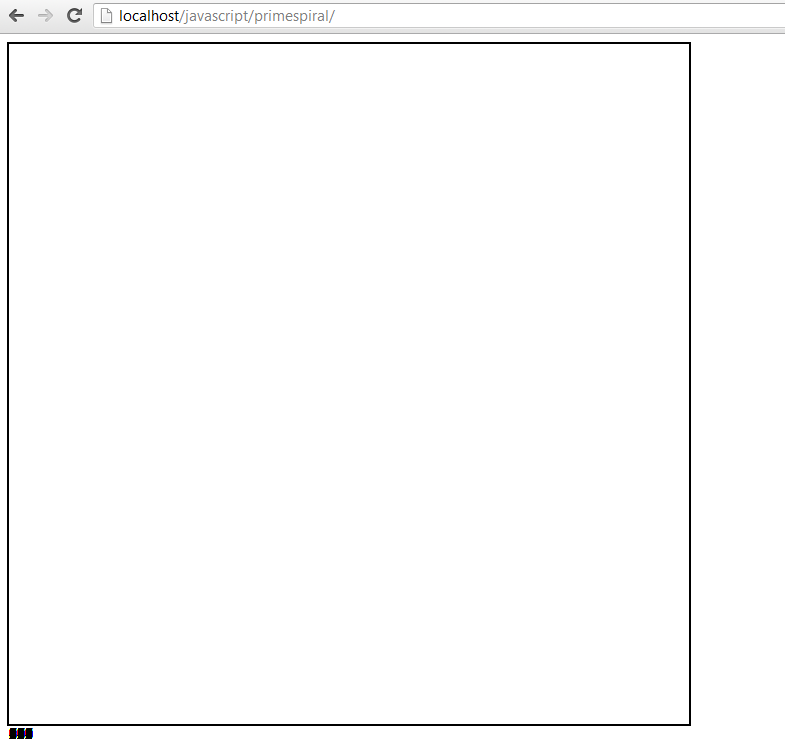Ooops! Something went wrong. I scanned through my code, and luckily I spotted my mistake quickly. I had included an extra “s” in my polar coordinates to Cartesian coordinates conversion function. Here’s the updated output. This process of debugging a mistake can sometimes be quite frustrating, so it requires developing patience.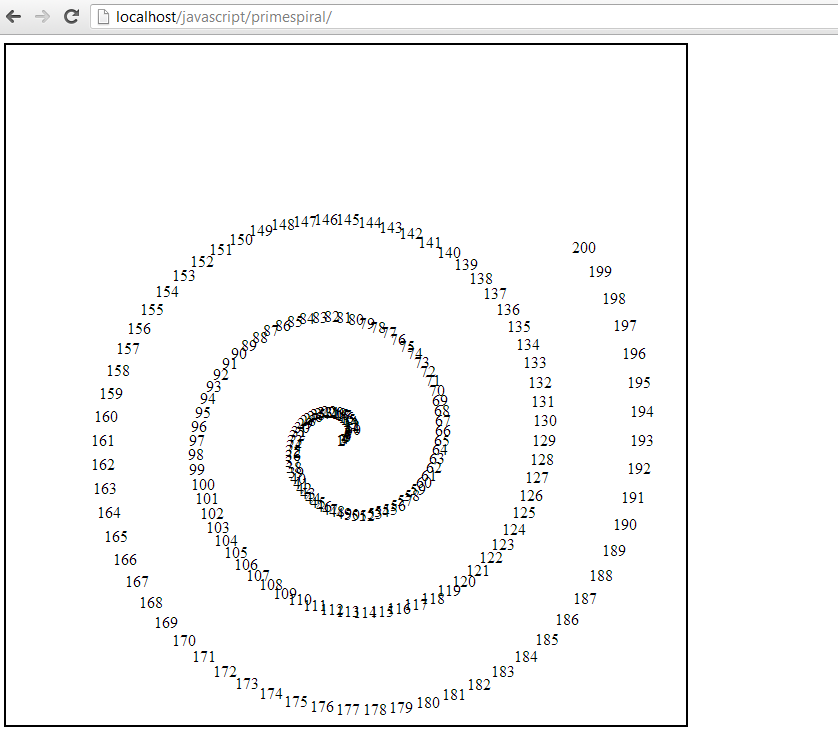It is clear that my basic concept works at this stage. The numbers look very tightly jumbled at the beginning, which is probably because the angle rotation isn’t sufficient early on. My assumption that a linear increase in angle would work is probably wrong. Also, the spiral isn’t centred inside the canvas, suggesting that I will want to work on my conversion function.

I want the angles to start spread out more than they are, and then slowly increase over time. I tried some of the following, none of which produced the desired effect.

// tried varying the fraction increase of the angle
theta = (index – 1) * (1 / 16) * Math.PI;

// tried using a reciprocal function for the angle
theta = (index – 1) * (10 / index) * Math.PI;

// tried using a reciprocal square root function for the angle
theta = (index – 1) * (10 / Math.sqrt(index)) * Math.PI;

// trying a decay function on the angle
theta = (index – 1) * (Math.exp(-1*index/100)) * Math.PI;

I realized at this stage that what I wanted was not a constant increase in the angle, but a constant distance between the points, after their conversion into Cartesian coordinates. I decided to try and draw this out on paper to see if I could work out an algebraic relationship. I decided then to draw the following diagram to see if it would give me any insights on how to proceed: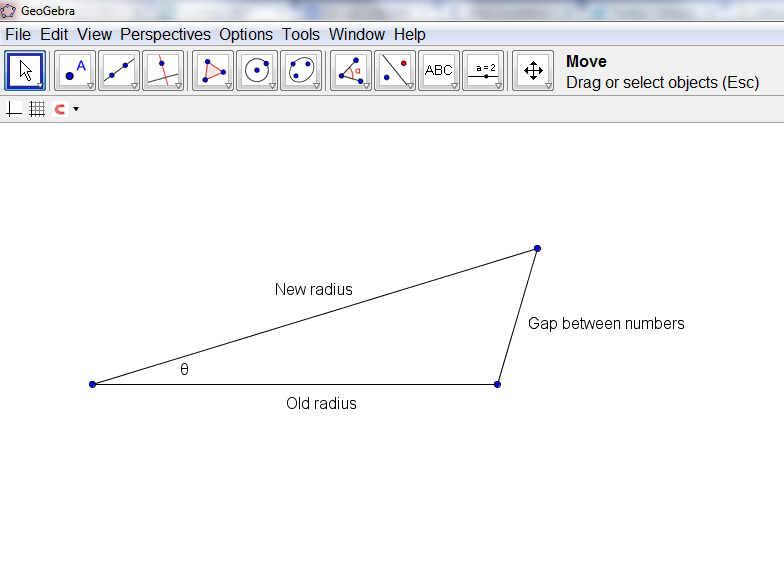When I looked at this, I realized that I could use the Cosine law to find the angle, given the new radius, old radius, and the gap between the letters. Initially, I tried a constant gap between the numbers, but after some playing around, I decided to gradually increase the font size between the numbers, and at the same time, gradually increase the gap between the letters. I ended up settling down on this as my final set of formulas for determining the position of the numbers.

// draw the spiral of numbers
function drawNumbers() {

// define the variables

// cycle through each number from 1 to the maximum number of numbers given
for (var index = 1; index <= primespiral.settings.numberOfNumbers; index++) { // set the radius so that it increases as the index increases, but is initially zero // note that we also want to scale the radius down slightly so that our spiral // is slightly smaller than the canvas radius = (index - 1) / primespiral.settings.numberOfNumbers * (primespiral.settings.dimension / 2) * 0.90 + 10; // keep track of the old radius radiusnew = (index) / primespiral.settings.numberOfNumbers * (primespiral.settings.dimension / 2) * 0.90 + 10; // fontsize should increase linearly as the index does fontSize = Math.round((index) / primespiral.settings.numberOfNumbers * 10 + 10); // calculate the rhs of the cosine law rhs = (radius*radius + radiusnew*radiusnew - (fontSize*1.5)*(fontSize*1.5))/(2*radius*radiusnew); // ensure that there is a minimum radius if (rhs < 0) { rhs = 0.1; } // our angle should increase such that the distance between the numbers is kept constant; theta = Math.acos(rhs); // now convert these into Cartesian coordinates coordinates = convertFromPolarToCartesian(radius, primespiral.currentAngle); // increment the next angle primespiral.currentAngle += theta; // and then actually draw this number drawNumber(index, coordinates.x, coordinates.y, fontSize); } }

How this works is that it calculates the current radius, the radius of where the next number will be placed, and then calculates the angle that corresponds to the gap between the letters, and then it saves all of this information for the next iteration of the loop by incrementing the current angle variable. I also worked on the portion of my script that converts the polar coordinates into Cartesian coordinates in an effort to better centre the spiral. This is my output with this code: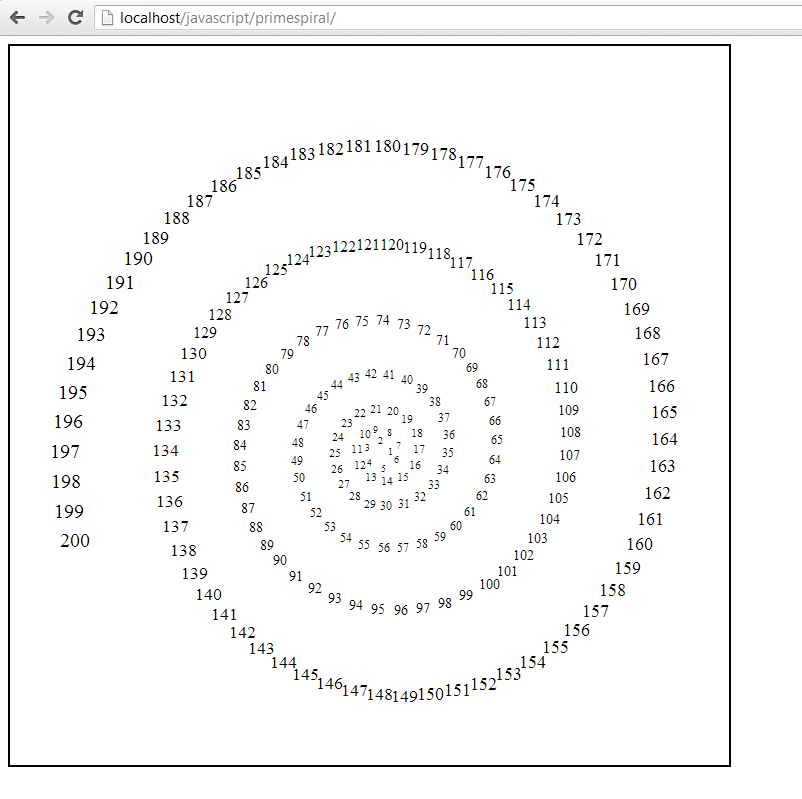I decided, somewhat arbitrarily, that the next step is to identify which of the numbers are prime, and highlight them in some way to make them distinct. This means that I needed to write a function to determine if a given number is prime, and then pass along this information to the function that draws the numbers. Here was what I ended up with. Note that this function actually doesn’t work completely (except for “small” numbers) as noted in the comment at the end.

// identifies if a given number is prime or not
function isPrime(number) {
// naive algorithm
var primes = new Array(
2,3,5,7,11,13,17,19,23,29,
31,37,41,43,47,53,59,61,67,71,
73,79,83,89,97,101,103,107,109,113,
127,131,137,139,149,151,157,163,167,173,
179,181,191,193,197,199,211,223,227,229,
233,239,241,251,257,263,269,271,277,281,
283,293,307,311,313,317,331,337,347,349,
353,359,367,373,379,383,389,397,401,409,
419,421,431,433,439,443,449,457,461,463,
467,479,487,491,499,503,509,521,523,541,
547,557,563,569,571,577,587,593,599,601,
607,613,617,619,631,641,643,647,653,659,
661,673,677,683,691,701,709,719,727,733,
739,743,751,757,761,769,773,787,797,809,
811,821,823,827,829,839,853,857,859,863,
877,881,883,887,907,911,919,929,937,941,
947,953,967,971,977,983,991,997,1009,1013);

// check to see if the number is 1
if (number == 1) {
return false;
}

// first check to see if our potential prime is in the list
for (var i = 0; i < primes.length; i++) { if (primes[i] == number) { return true; } } // now check every prime smaller than n for (i = primes.length - 1; i > 0; i–) {
if (number % primes[i] == 0) {
return false;
}
}

// this isn’t actually guaranteed, unless n < 1013*1013 return true; }

Putting all of this together, and I end up with this. You can view the entire JavaScript file here.

What mathematics did I end up using? Do you think that this kind of application of mathematics would be a valuable one for students to experience?

I’ve created a video summary of the workshop that I facilitated recently on math in the real world. You can watch it below.

My presentation slides for the original workshop are here.

I went to the playground today with my son, and realized that the equipment at a playground could be really useful as apparatus for scientific experiments. Here are a few demonstrations of scientific principles:

How would you turn these demonstrations into experiments? What kind of data would you collect? What are some other examples of playground physics?